# Power Converter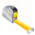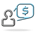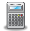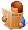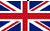Converter Currencies Calculator Articles

 Other units Area Energy Flowrate Force Length Mass Power Pressure Velocity Volume Temperature Time ______________ AcresAresBars Barrels per day Baryes BTUs BTUs per hour BTUs per minute BTUs per second Centimeters (cm) cm/sec Chains Cooking measures Crinals Cubic centimeters (cc) cc/sec cc-atmosphere Cubic decimeters Cubic feet Cubic feet per minute Cubic feet per second Cubic inches Cubic inches per minute Cubic inches per second Cubic meters Cubic meters per hour Cubic yards Cubic yards per minute Decimeters (dm) Drams DynesErgs per second Fluid drachmas Fluid ounces (fl.oz.) Feet (ft) Feet per hour Feet per minute Feet per second ft-lbf ft-lbf/hour ft-lbf/min ft-lbf/sec Furlongs Gallons Gallons per day Gallons per minute Gallons per second Gigawatts (GW) Gills Grains Grams (g) Gram-calories (g-cal) Hectares Hectometers Hundredweights (cwt) Inches (in) Inches H2O Inches Hg Inch-pound-forces (in-lbf) IST calories Joules (J) Joules per second (J/sec) Kilocalories (kcal) Kilograms (kg) kg-meters/sq. sec Kilogram-calories Kilogram-forces (kgf) Kgf/sq. cm Kgf/sq. meter Kilometers (km) Kilometers per hour (km/h) KiloPascal(kPa) Kilowatts (kW) Kilowatts per hour (kWh) Kips Knots Liters (l) Liters per minute Liters per second Long tons (imperial tons) MegaPascal(mPa) Megawatts (MW) Meters (m) Meters per minute Meters per second Metric horsepower Miles Miles per hour (mph) Miles per minute Milligrams (mg) Milliliters (ml) Millimeters (mm) Miner inches Newton (N) Ounces (oz) Ounce-force Pascal (Pa) Pints Pounds (lb) Pounds/sq. foot Pounds/sq. inch Poundals Poundals/sq. foot Poundals/sq. inch Pound-CHU Pound-force (lbf) Quarts Quarters Rods Shoe size Short tons/sq. footSlugs Square centimeters Square decimeters Square feet Square kilometers Square meters Square miles Square millimeters Square yards Stones Thermocalories TonsTons (refrigeration) Tonnes (metric tons) Torrs U.S. gallons U.S. horsepower per hour U.S. tons Watts Watts per hour Yards ________________ Ancient Greek: area Ancient Greek: currency Ancient Greek: length Ancient Greek: mass Ancient Greek: volume Ancient Roman: area Ancient Roman: length Ancient Roman: mass/coin Ancient Roman: volume Apothecary: imperial UK Apothecary: US/UK Apothecary: weightLeague units ________________ Calculator Contact Main website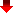Converting power units is an important process in various industries such as energy, manufacturing, and transportation. It helps to ensure accurate measurements and comparisons between different systems.

In the energy sector, for instance, converting power units can provide an accurate estimate of the energy consumption of a system, which is crucial for energy efficiency and cost analysis. In manufacturing, converting power units helps to measure the output of machines, which is important for production planning and optimization.

In the transportation industry, converting power units helps to measure the engine power of vehicles, which is essential for determining their performance and efficiency. Converting power units also ensures that data is comparable between different regions and countries, which helps to facilitate international trade and cooperation.

Furthermore, converting power units is necessary for the accurate design of systems, as well as for the accurate calculation of system performance, maintenance, and repair costs. For example, converting from kilowatts (kW) to megawatts (MW) can provide a more comprehensive understanding of the total energy consumption of a city. Another example is converting from horsepower (HP) to kilowatts (kW), which is useful for comparing the engine power of different vehicles.

MetricToImperial.com